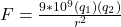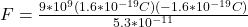## Calculate the electric field acting on the electron in a hydrogen atom due to the proton in the nucleus the radius of the orbit of the elect

Question

Calculate the electric field acting on the electron in a hydrogen atom due to the proton in the nucleus the radius of the orbit of the electron is 5.3×10^-11

in progress 0
6 months 2021-07-14T08:06:07+00:00 1 Answers 33 views 0

## Answers ( )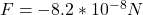Explanation:

From the question we are told that:

Radius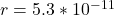Where

Proton Charge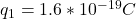Charge of Electron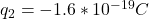Generally the equation for Coulomb’s Law is mathematically given by